# 重要技巧

1. 使用 x & 1 == 1 判断奇偶数。（注意，一些编辑器底层会把用%判断奇偶数的代码，自动优化成位运算）

2. 不使用第三个数，交换两个数。x = x ^ y ， y = x ^ y ， x = x ^ y。（早些年喜欢问到，现在如果谁再问，大家会觉得很low）

3. 两个相同的数异或的结果是 0，一个数和 0 异或的结果是它本身。（对于找数这块，异或往往有一些别样的用处。）

4. x & (x – 1) ，可以将最右边的 1 设置为 0。（这个技巧可以用来检测 2的幂，或者检测一个整数二进制中 1 的个数，又或者别人问你一个数变成另一个数其中改变了多少个bit位，统统都是它）

5. i+(~i)=-1，i 取反再与 i 相加，相当于把所有二进制位设为1，其十进制结果为-1。

6. 对于int32而言，使用 n >> 31取得 n 的正负号。并且可以通过 (n ^ (n >> 31)) – (n >> 31) 来得到绝对值。（n为正，n >> 31 的所有位等于0。若n为负数，n >> 31 的所有位等于1，其值等于-1）

7. 使用 (x ^ y) >= 0 来判断符号是否相同。（如果两个数都是正数,则二进制的第一位均为0,x^y=0；如果两个数都是负数,则二进制的第一位均为1；x^y=0 如果两个数符号相反,则二进制的第一位相反,x^y=1。有0的情况例外，^相同得0，不同得1）

8. “异或”是一个无进位加法，说白了就是把进位砍掉。比如01^01=00。

9. “与”可以用来获取进位，比如01&01=01，然后再把结果左移一位，就可以获取进位结果。

# 使用掩码遍历二进制位

\[0000\ 0000\ 0000\ 0000\ 0000\ 0000\ 0000\ 0001\]
0000 0000 0000 0000 0000 0000 0000 0001

\[0000\ 0000\ 0000\ 0000\ 0000\ 0000\ 0000\ 0010\]
0000 0000 0000 0000 0000 0000 0000 0010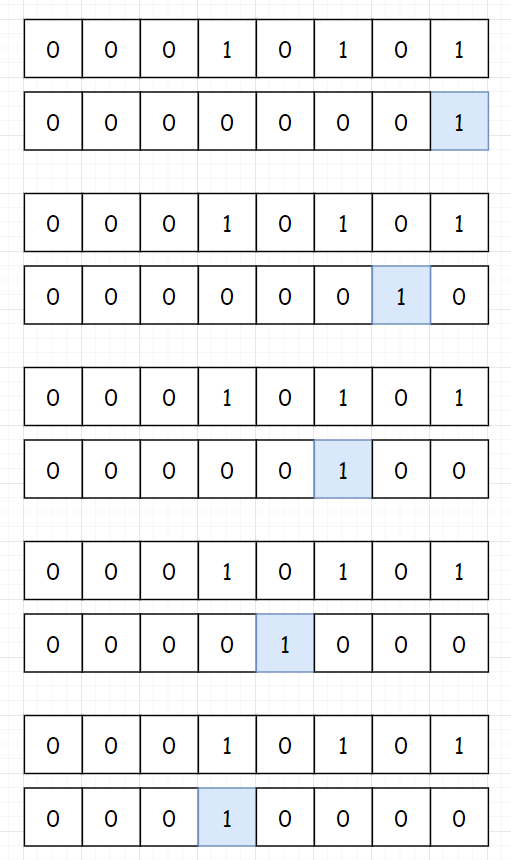Java

``````public int hammingWeight(int n) {
int bits = 0; // 用来储存 1 的个数

int mask = 1;  // 掩码，从最低位开始

for (int i = 0; i < 32; i++) {
// 注意这里是不等于0，而不是等于1，因为我们的位数是不断在变化的，可能等于2、4、8...
// 使用按位操作符时要注意，相等（==）与不相等（!=）的优先级在按位运算符之上！！！！
// 使用按位运算符时，最好加上括号()
if ((n & mask) != 0) {
bits++;
}
}
return bits;
}``````

# 无符号右移遍历二进制位

• \(n \& 1 = 0\)，则 n 二进制 最右一位 为 00 ；
• \(n \& 1 = 1\)，则 n 二进制 最右一位 为 11 。

1. 判断 n 最右一位是否为 1 ，根据结果计数。
2. 将 n 右移一位（本题要求把数字 n 看作无符号数，因此使用 无符号右移 操作）。

1. 初始化数量统计变量 res = 0。
2. 循环逐位判断： 当 n = 0 时跳出。
3. `res += n & 1` ： 若 \(n \& 1 = 1\) ，则统计数 res 加一。
4. `n >>= 1` ： 将二进制数字 n 无符号右移一位（ Java 中无符号右移为 “>>>” ） 。
5. 返回统计数量 res。
``````public class Solution {
public int hammingWeight(int n) {
int res = 0;
while(n != 0) {
res += n & 1;  // 遍历
n >>>= 1;  // 无符号右移
}
return res;
}
}``````

# 反转最后一个 1

\((n – 1)\) 解析： 二进制数字 n 最右边的 1 变成 0 ，此 1 右边的 0 都变成 1 。
\(n \& (n – 1)\) 解析： 二进制数字 n 最右边的 1 变成 0 ，其余不变。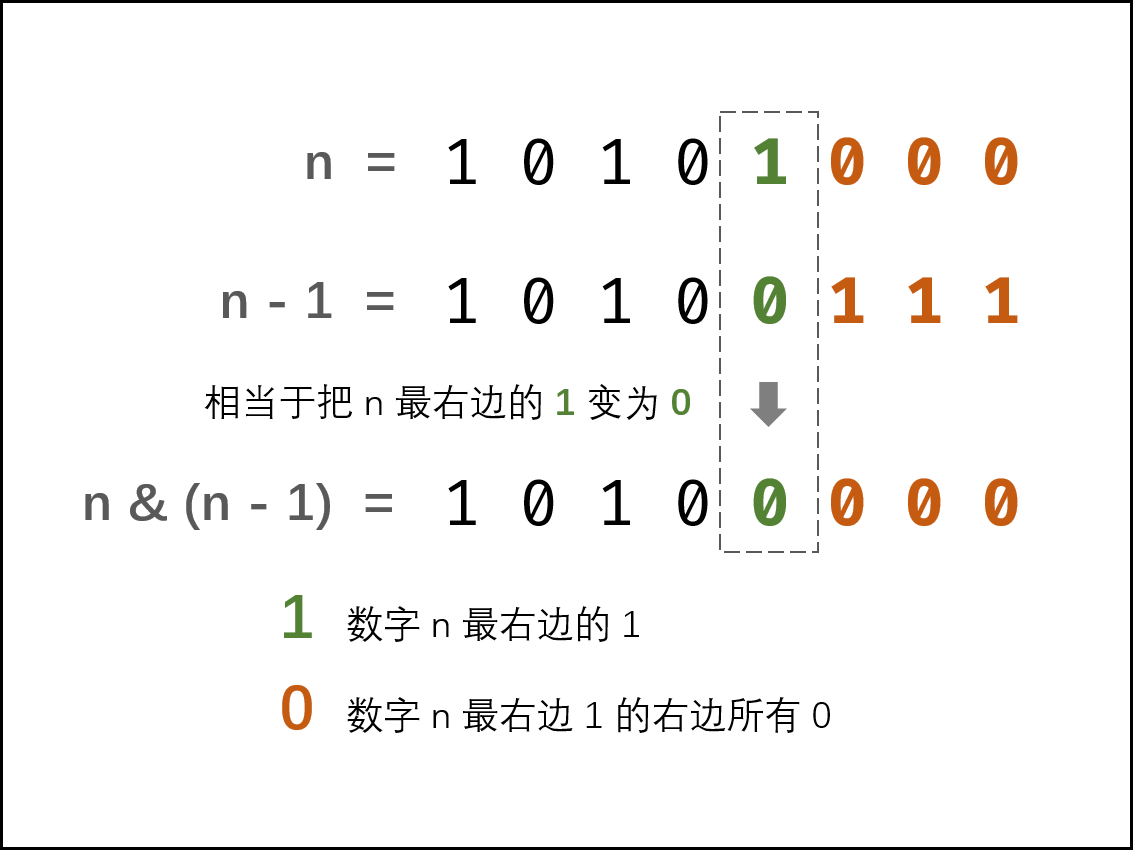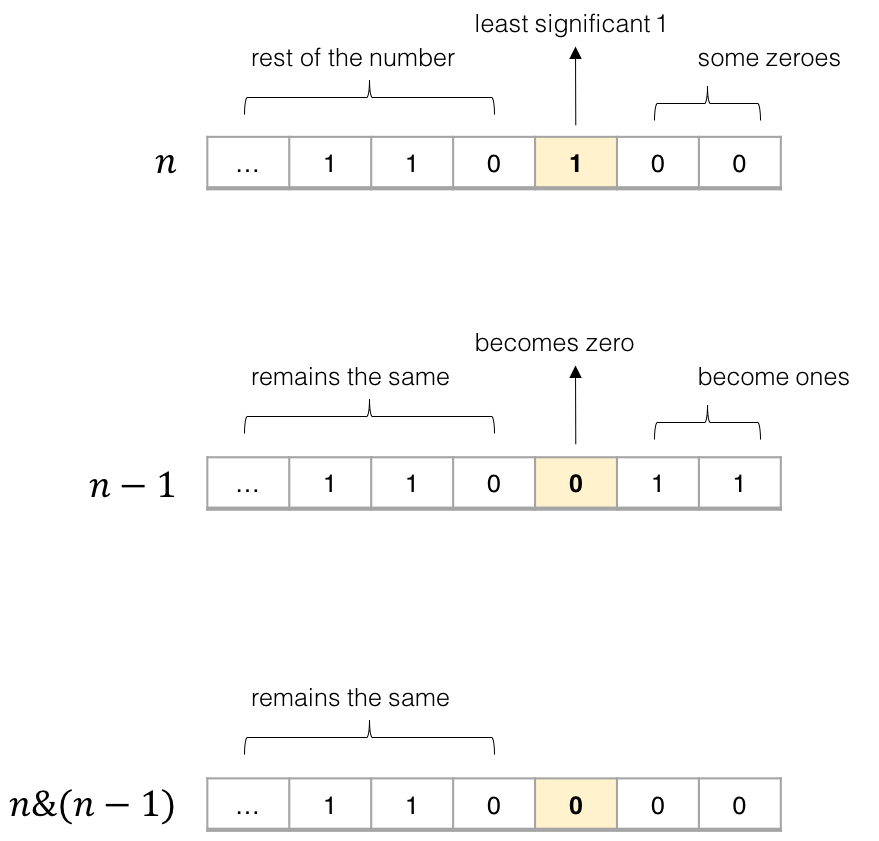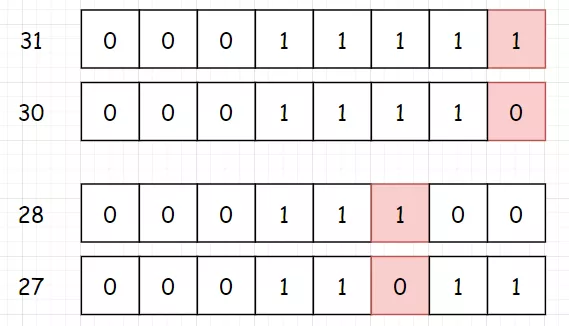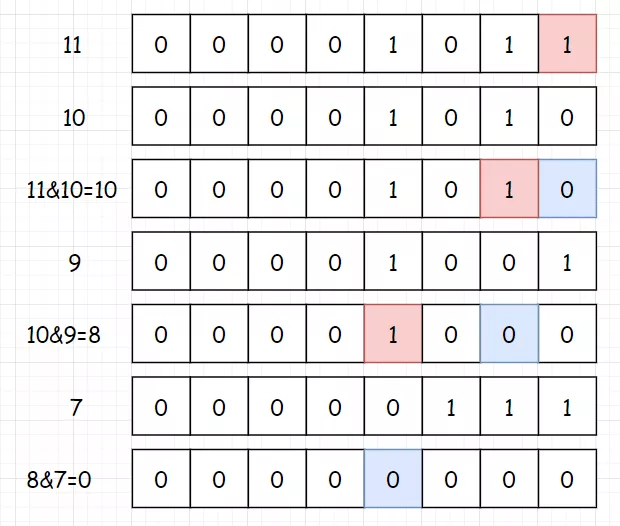Java

``````public int hammingWeight(int n) {

int sum = 0;  // 用来存放 1 的个数

while (n != 0) {
sum++;
n &= (n - 1);
}
return sum;
}``````

# Java内置函数：1 的个数

``````public int hammingWeight(int n) {
return Integer.bitCount(n);
}``````

# 异或相消（异或运算的特性）

• 交换律：\(p \oplus q = q \oplus p\)
• 结合律：\(p \oplus (q \oplus r) = (p \oplus q) \oplus r\)
• 恒等率：\(p \oplus 0 = p\)
• 归零率：\(p \oplus p = 0\)

1. 任何数和 0 做异或运算，结果仍然是原来的数，即 \(a \oplus 0=a\)
2. 任何数和其自身做异或运算，结果是 0，即 \(a \oplus a=0\)
3. 异或运算满足交换律和结合律，即 \(a \oplus b \oplus a=b \oplus a \oplus a=b \oplus (a \oplus a)=b \oplus0=b\)

\[(a_{1} \oplus a_{1}) \oplus (a_{2} \oplus a_{2}) \oplus \cdots \oplus (a_{m} \oplus a_{m}) \oplus a_{m+1}\]

\[0 \oplus 0 \oplus \cdots \oplus 0 \oplus a_{m+1}=a_{m+1}\]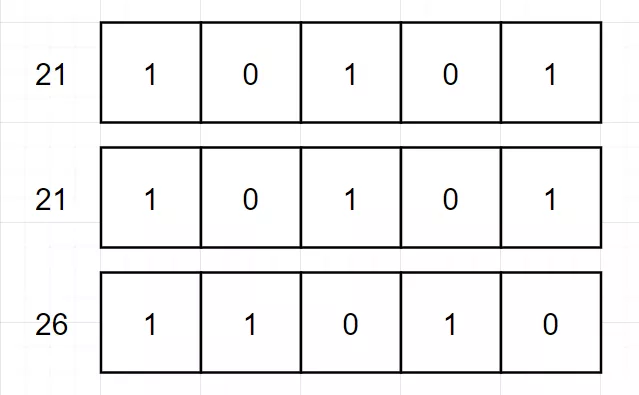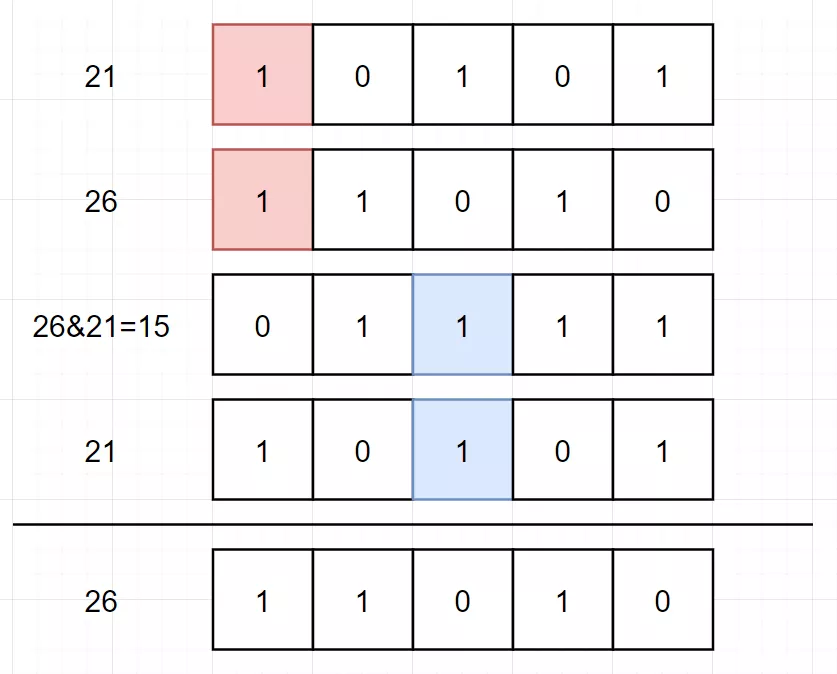Java

``````class Solution {
public int singleNumber(int[] nums) {
int single = 0;
for (int num : nums) {
single ^= num;
}
return single;
}
}``````

# 实例

## 位1的个数

• 请注意，在某些语言（如 Java）中，没有无符号整数类型。在这种情况下，输入和输出都将被指定为有符号整数类型，并且不应影响您的实现，因为无论整数是有符号的还是无符号的，其内部的二进制表示形式都是相同的。
• 在 Java 中，编译器使用二进制补码记法来表示有符号整数。因此，在上面的 示例 3 中，输入表示有符号整数 -3。

• 如果多次调用这个函数，你将如何优化你的算法？

``````输入：00000000000000000000000000001011

``````输入：00000000000000000000000010000000

``````输入：11111111111111111111111111111101

## 答案

### 方法 1：循环和位移动

\[0000\ 0000\ 0000\ 0000\ 0000\ 0000\ 0000\ 0001\]
0000 0000 0000 0000 0000 0000 0000 0001

\[0000\ 0000\ 0000\ 0000\ 0000\ 0000\ 0000\ 0010\]
0000 0000 0000 0000 0000 0000 0000 0010

Java

``````public int hammingWeight(int n) {
int bits = 0;
for (int i = 0; i < 32; i++) {
if ((n & mask) != 0) {
bits++;
}
}
return bits;
}``````

• 时间复杂度：\(O(1)\)。运行时间依赖于数字 n 的位数。由于这题中 n 是一个 32 位数，所以运行时间是 \(O(1)\) 的。

• 空间复杂度：\(O(1)\)。没有使用额外空间。

• \(n \& 1 = 0\)，则 n 二进制 最右一位 为 00 ；
• \(n \& 1 = 1\)，则 n 二进制 最右一位 为 11 。

1. 判断 n 最右一位是否为 1 ，根据结果计数。
2. 将 n 右移一位（本题要求把数字 n 看作无符号数，因此使用 无符号右移 操作）。

1. 初始化数量统计变量 res = 0。
2. 循环逐位判断： 当 n = 0 时跳出。
3. `res += n & 1` ： 若 \(n \& 1 = 1\) ，则统计数 res 加一。
4. `n >>= 1` ： 将二进制数字 n 无符号右移一位（ Java 中无符号右移为 “>>>” ） 。
5. 返回统计数量 res。
``````public class Solution {
public int hammingWeight(int n) {
int res = 0;
while(n != 0) {
res += n & 1;  // 遍历
n >>>= 1;  // 无符号右移
}
return res;
}
}``````

### 方法 2：位操作的小技巧

\((n – 1)\) 解析： 二进制数字 n 最右边的 1 变成 0 ，此 1 右边的 0 都变成 1 。
\(n \& (n – 1)\) 解析： 二进制数字 n 最右边的 1 变成 0 ，其余不变。Java

``````public int hammingWeight(int n) {
int sum = 0;
while (n != 0) {
sum++;
n &= (n - 1);
}
return sum;
}``````

• 时间复杂度：\(O(1)\)。运行时间与 n 中位为 1 的有关。在最坏情况下，n 中所有位都是 1 。对于 32 位整数，运行时间是 \(O(1)\) 的。

• 空间复杂度：\(O(1)\)。没有使用额外空间。

## 只出现一次的数字

``````输入: [2,2,1]

``````输入: [4,1,2,1,2]

### 答案

• 使用集合存储数字。遍历数组中的每个数字，如果集合中没有该数字，则将该数字加入集合，如果集合中已经有该数字，则将该数字从集合中删除，最后剩下的数字就是只出现一次的数字。

• 使用哈希表存储每个数字和该数字出现的次数。遍历数组即可得到每个数字出现的次数，并更新哈希表，最后遍历哈希表，得到只出现一次的数字。

• 使用集合存储数组中出现的所有数字，并计算数组中的元素之和。由于集合保证元素无重复，因此计算集合中的所有元素之和的两倍，即为每个元素出现两次的情况下的元素之和。由于数组中只有一个元素出现一次，其余元素都出现两次，因此用集合中的元素之和的两倍减去数组中的元素之和，剩下的数就是数组中只出现一次的数字。

\[(a_{1} \oplus a_{1}) \oplus (a_{2} \oplus a_{2}) \oplus \cdots \oplus (a_{m} \oplus a_{m}) \oplus a_{m+1}\]

\[0 \oplus 0 \oplus \cdots \oplus 0 \oplus a_{m+1}=a_{m+1}\]

Java

``````class Solution {
public int singleNumber(int[] nums) {
int single = 0;
for (int num : nums) {
single ^= num;
}
return single;
}
}``````

• 时间复杂度：\(O(n)\)，其中 n 是数组长度。只需要对数组遍历一次。

• 空间复杂度：\(O(1)\)

## 剑指 Offer 56 – II. 数组中数字出现的次数 II

``````输入：nums = [3,4,3,3]

``````输入：nums = [9,1,7,9,7,9,7]

### 位运算答案

``````// 虽然位运算很麻烦，但是我也得试试啊
class Solution {
public int singleNumber(int[] nums) {

// 位运算有点麻烦

// 把所有数的二进制位相加，对每一位取余3，那么剩下来的就是我们需要找的数字了
int[] counts = new int; // 用来存储答案数字的32位二进制

// 遍历数组中的所有数，将他们的二进制位相加，存起来
for(int num : nums) {
// 从0到31，从低位到高位
for(int j = 0; j < 32; j++) {
counts[j] += num & 1;
num >>>= 1;
}
}

// 从高位开始，把每一位二进制 % 3
int res = 0;    // 结果答案
for(int i = 31; i >= 0; i--) {
// 先移位再加个位，就如 sum += sum * 10 + 个位
res <<= 1;
res |= counts[i] % 3;
}
return res;

}
}``````

### 哈希表答案

``````class Solution {
public int singleNumber(int[] nums) {

// 位运算有点麻烦

// 哈希表
Map<Integer, Integer> map = new HashMap<>();

for (int i = 0; i < nums.length; i++) {

int count = map.getOrDefault(nums[i], 0) + 1;

map.put(nums[i], count);
}

for (int i = 0; i < nums.length; i++) {

int count = map.getOrDefault(nums[i], 0);
if (count == 1) {
return nums[i];
}
}

return 0;
}
}``````

## 两数之和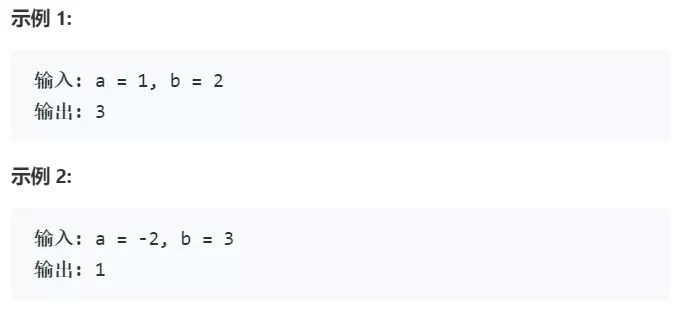## 答案

• “异或”是一个无进位加法，说白了就是把进位砍掉。比如01^01=00。

• “与”可以用来获取进位，比如01&01=01，然后再把结果左移一位，就可以获取进位结果。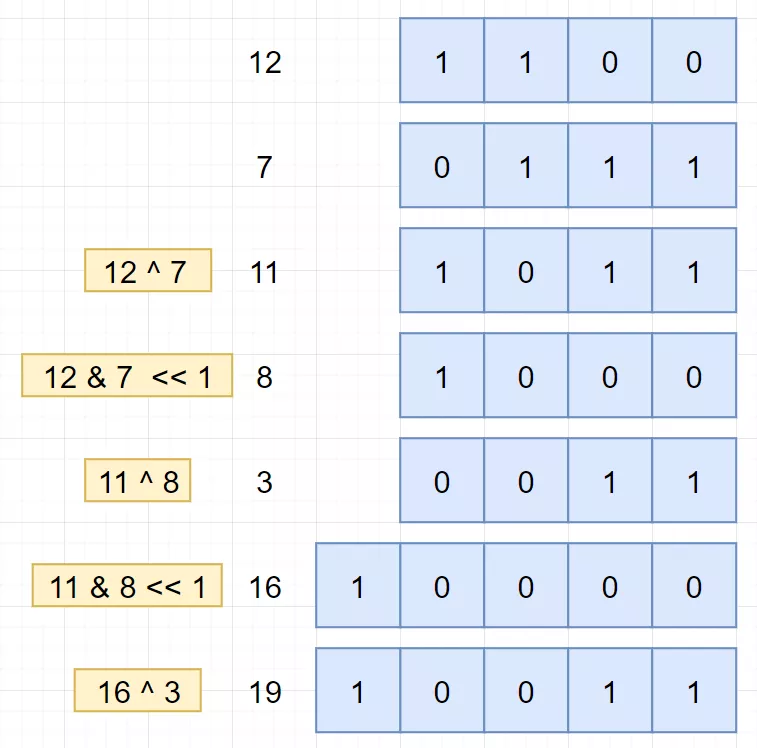``````//JAVA
class Solution {
public int getSum(int a, int b){
while(b != 0){
int temp = a ^ b;
b = (a & b) << 1;
a = temp;
}
return a;
}
}``````

## 剑指 Offer 65. 不用加减乘除做加法

``````输入: a = 1, b = 1

### 答案

``````//JAVA
class Solution {
public int add(int a, int b) {
// 该位都为1，&，则进位
// 异或运算，^，非进位加

// 我们使用temp来记录进位的位数二进制
// 每次我们都将 非进位和 与 进位二进制 做非进位加法运算，直到没有进位为止（进位为0）
while(b != 0) { // 当进位为 0 时跳出
int temp = (a & b) << 1;  // temp = 进位
a ^= b; // a = 非进位和
b = temp; // b = 进位
}
return a;
}
}``````
Tags: